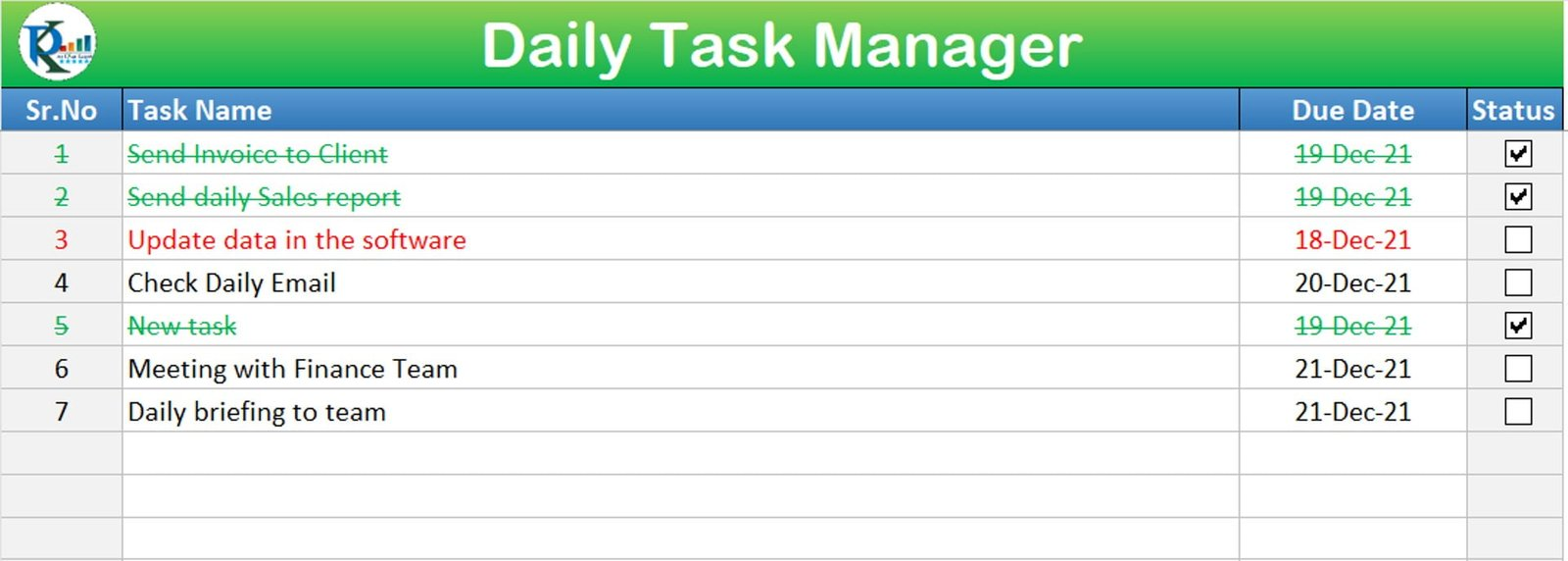# Automated Daily Task Manager with auto Check boxes

Daily Task Manager Template is a very useful template to manage your daily tasks. We have used VBA automation to create an auto checkbox when the new task will be entered. We have used conditional formatting to show the completed task and overdue task. The status check box will be deleted automatically when you will delete a task

### Fully Functional To-do List in ExcelTo create and delete a check box automatically, we have used below given VBA Code on the worksheet change event-

```Private Sub Worksheet_Change(ByVal Target As Range)

Dim check_box
Dim rng As Range
Dim chk_rng As Range

Dim sp As Shape

For Each rng In Target
Set chk_rng = Cells(rng.Row, 4)
If rng.Column = 2 And rng.Row > 2 Then
If rng.Value <> "" Then
If chk_rng.Value = "" Then
Set check_box = Sheet2.CheckBoxes.Add(chk_rng.Left + 10, chk_rng.Top - 2, 40, 20)
check_box.Characters.Text = ""
chk_rng.Value = "False"
chk_rng.NumberFormat = ";;;"
End If
End If

If rng.Value = "" Then
For Each sp In ActiveSheet.Shapes
sp.Delete
chk_rng.Value = ""
End If
Next
End If

End If
Next

End Sub```